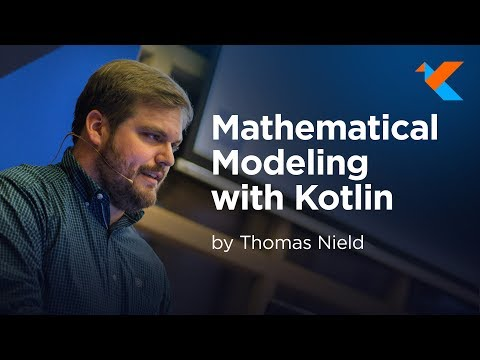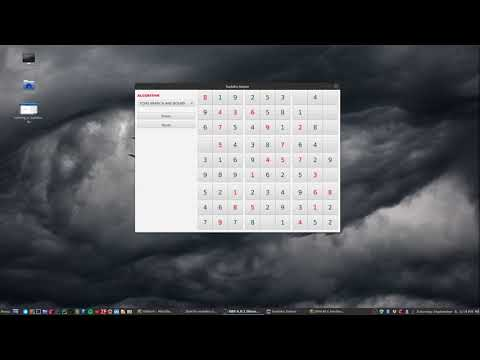A Simple Neural NetworkKotlinConf 2018 - Mathematical ModelingCreating a Sudoku Solver from ScratchTraveling Salesman ProblemText Categorization w/ Naive BayesMonty Hall ProblemSolving World's Hardest Sudoku

## Friday, November 6, 2015

### RxJava- Flatmap Filtering

After doing RxJava for several months, I have found the key to making it extra useful is not just knowing the operators, but knowing how to combine them together. The built-in operators can be cleverly composed together with other operators to create new operations, like flatMap() and subscribeOn() to achieve parallelization.

When I started mixing object-oriented and reactive programming together, I came across some interesting problems and design decisions. If I have a class that contained one or more Observable properties, I was not quite sure how to filter on these properties.

For instance, let's say you have a type called Transaction. It has four properties that you typically might expect on a bank transaction object.
```public interface Transaction {
int getID();
LocalDate getDate();
BigDecimal getAmount();
Observable<BigDecimal> getBalance();
}
```
Suppose we have an Observable streaming these Transaction objects from some IO source or a reactive JDBC library. If wanted to filter these emissions to just today's date, that is easy enough using the filter() operator. It only passes emissions that meet the boolean condition specified by a lambda.
`Observable<Transaction> transactions = importFromDb();`
```
Observable<Transaction> todaysTransactions = transactions
.filter(t -> t.getDate().equals(LocalDate.now()));

todaysTransactions.subscribe(System.out::println);
```
Easy enough, right? But now what if we wanted to filter on the Observable property getBalance()? This property will dynamically push a BigDecimal value reflecting the bank balance after that transaction took place. Say we want to filter the stream of transactions to where the balance is less than \$100. The problem is we can't use the filter operator thanks to the monad. Try it. You will never be able to compile because you will always return an Observable, not a boolean which the filter() operator expects.
`Observable<Transaction> transactions = importFromDb();`
```
Observable<Transaction> underHundred = transactions
.filter(t -> t.getBalance()
.map(d -> d.compareTo(BigDecimal.valueOf(100)) < 0)); //argh!!!

underHundred.subscribe(System.out::println);
```
The problem is we are passing the filter() operator an Observable<Boolean>, not a boolean which it needs. We could call toBlocking() and extract that boolean value but that will break the monad (which is bad).

So how do we filter on this Observable property? I learned through experimentation the flatMap() has a lot of tricks up its sleeves, and we can use it filter on an Observable property on a stream of objects.
```Observable transactions = importFromDb();

Observable<Transaction> underHundred = transactions
.flatMap(t -> t.getBalance()
.filter(b -> b.compareTo(BigDecimal.valueOf(100)) < 0)
.map(b -> t)
);

underHundred.subscribe(System.out::println);
```
Study the code above very closely and notice everything that happened inside that flatMap(). For each Transaction, we called the getBalance() which emits the balance. But we immediately filtered  where the balance is less than 100. Any balance emissions that pass this condition are then mapped back to the original Transaction item they came from using .map(b -> t).  This Transaction is emitted out of the flatMap(), but Transactions that failed to meet the criteria will come out as empty Observables.

This is how you can filter on Observable properties. Of course, the above example assumes the getBalance() method returns a single emission followed by an onCompleted() call. You might encounter scenarios where you need to filter on a property that emits multiple values, and this can be very useful if that is your intention. You can emit multiple conditions from a property, and those that pass will emit the item through while the conditions that fail do not.

Let me know if you have any questions and make it a great day!

#### 1 comment:

1.Great Content, Just loved the way you presented it… Keep up the good work Brown Cow Skin Leather Long Coat